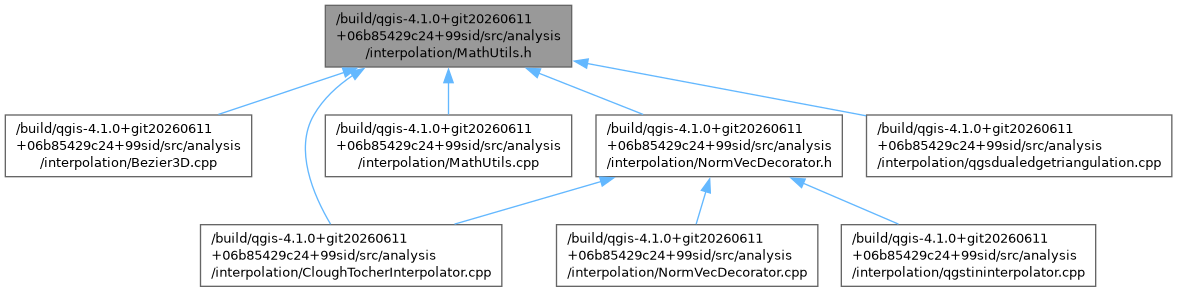QGIS API Documentation  3.23.0-Master (b5237dafc3)
MathUtils.h File Reference
#include <cmath>
#include "qgis_analysis.h"
Include dependency graph for MathUtils.h:This graph shows which files directly or indirectly include this file:Go to the source code of this file.

MathUtils

Macros

#define SIP_NO_FILE

Functions

double ANALYSIS_EXPORT MathUtils::angle (QgsPoint *p1, QgsPoint *p2, QgsPoint *p3, QgsPoint *p4)
Calculates the angle between two segments (in 2 dimension, z-values are ignored) More...

bool ANALYSIS_EXPORT MathUtils::BarycentricToXY (double u, double v, double w, QgsPoint *p1, QgsPoint *p2, QgsPoint *p3, QgsPoint *result)

bool ANALYSIS_EXPORT MathUtils::calcBarycentricCoordinates (double x, double y, QgsPoint *p1, QgsPoint *p2, QgsPoint *p3, QgsPoint *result)
Calculates the barycentric coordinates of a point (x,y) with respect to p1, p2, p3 and stores the three barycentric coordinates in 'result'. Thus the u-coordinate is stored in result::x, the v-coordinate in result::y and the w-coordinate in result::z. Attention: p1, p2 and p3 have to be ordered counterclockwise. More...

double ANALYSIS_EXPORT MathUtils::calcBernsteinPoly (int n, int i, double t)
Calculates the value of a Bernstein polynomial. More...

double ANALYSIS_EXPORT MathUtils::calcCubicHermitePoly (int n, int i, double t)
Calculates the value of a cubic Hermite polynomial. More...

double ANALYSIS_EXPORT MathUtils::cFDerBernsteinPoly (int n, int i, double t)
Calculates the first derivative of a Bernstein polynomial with respect to the parameter t. More...

double ANALYSIS_EXPORT MathUtils::cFDerCubicHermitePoly (int n, int i, double t)
Calculates the first derivative of a cubic Hermite polynomial with respect to the parameter t. More...

bool ANALYSIS_EXPORT MathUtils::circumcenter (QgsPoint *p1, QgsPoint *p2, QgsPoint *p3, QgsPoint *result)
Calculates the center of the circle passing through p1, p2 and p3. Returns true in case of success and false otherwise (e.g. all three points on a line) More...

double ANALYSIS_EXPORT MathUtils::crossVec (QgsPoint *first, Vector3D *vec1, QgsPoint *second, Vector3D *vec2)
Calculates the intersection of the two vectors vec1 and vec2, which start at first(vec1) and second(vec2) end. The return value is t2(multiplication of v2 with t2 and adding to 'second' results the intersection point) More...

bool ANALYSIS_EXPORT MathUtils::derVec (const Vector3D *v1, const Vector3D *v2, Vector3D *result, double x, double y)
Calculates the z-component of a vector with coordinates 'x' and 'y'which is in the same tangent plane as the tangent vectors 'v1' and 'v2'. The result is assigned to 'result'. More...

double ANALYSIS_EXPORT MathUtils::distPointFromLine (QgsPoint *thepoint, QgsPoint *p1, QgsPoint *p2)
Calculates the (2 dimensional) distance from 'thepoint' to the line defined by p1 and p2. More...

int ANALYSIS_EXPORT MathUtils::faculty (int n)
Faculty function. More...

bool ANALYSIS_EXPORT MathUtils::inCircle (QgsPoint *testp, QgsPoint *p1, QgsPoint *p2, QgsPoint *p3)
Tests, whether 'testp' is inside the circle through 'p1', 'p2' and 'p3'. More...

bool ANALYSIS_EXPORT MathUtils::inDiametral (QgsPoint *p1, QgsPoint *p2, QgsPoint *point)
Tests, whether 'point' is inside the diametral circle through 'p1' and 'p2'. More...

double ANALYSIS_EXPORT MathUtils::leftOf (const QgsPoint &thepoint, const QgsPoint *p1, const QgsPoint *p2)
Returns whether 'thepoint' is left or right of the line from 'p1' to 'p2'. Negative values mean left and positive values right. There may be numerical instabilities, so a threshold may be useful. More...

bool ANALYSIS_EXPORT MathUtils::lineIntersection (QgsPoint *p1, QgsPoint *p2, QgsPoint *p3, QgsPoint *p4)
Returns true, if line1 (p1 to p2) and line2 (p3 to p4) intersect. If the lines have an endpoint in common, false is returned. More...

bool ANALYSIS_EXPORT MathUtils::lineIntersection (QgsPoint *p1, QgsPoint *p2, QgsPoint *p3, QgsPoint *p4, QgsPoint *intersection_point)
Returns true, if line1 (p1 to p2) and line2 (p3 to p4) intersect. If the lines have an endpoint in common, false is returned. The intersecting point is stored in 'intersection_point. More...

int ANALYSIS_EXPORT MathUtils::lower (int n, int i)
Lower function. More...

void ANALYSIS_EXPORT MathUtils::normalFromPoints (QgsPoint *p1, QgsPoint *p2, QgsPoint *p3, Vector3D *vec)
Calculates the normal vector of the plane through the points p1, p2 and p3 and assigns the result to vec. If the points are ordered counterclockwise, the normal will have a positive z-coordinate;. More...

bool ANALYSIS_EXPORT MathUtils::normalLeft (Vector3D *v1, Vector3D *result, double length)
Assigns the vector 'result', which is normal to the vector 'v1', on the left side of v1 and has length 'length'. This method works only with two dimensions. More...

bool ANALYSIS_EXPORT MathUtils::normalMinDistance (Vector3D *tangent, Vector3D *target, Vector3D *result)
Calculates a Vector orthogonal to 'tangent' with length 1 and closest possible to result. Returns true in case of success and false otherwise. More...

bool ANALYSIS_EXPORT MathUtils::normalRight (Vector3D *v1, Vector3D *result, double length)
Assigns the vector 'result', which is normal to the vector 'v1', on the right side of v1 and has length 'length'. The calculation is only in two dimensions. More...

double ANALYSIS_EXPORT MathUtils::planeTest (QgsPoint *test, QgsPoint *pt1, QgsPoint *pt2, QgsPoint *pt3)
Tests, if 'test' is in the same plane as 'p1', 'p2' and 'p3' and returns the z-difference from the plane to 'test. More...

bool ANALYSIS_EXPORT MathUtils::pointInsideTriangle (double x, double y, QgsPoint *p1, QgsPoint *p2, QgsPoint *p3)
Returns true, if the point with coordinates x and y is inside (or at the edge) of the triangle p1,p2,p3 and false, if it is outside. p1, p2 and p3 have to be ordered counterclockwise. More...

double ANALYSIS_EXPORT MathUtils::triArea (QgsPoint *pa, QgsPoint *pb, QgsPoint *pc)
Returns the area of a triangle. If the points are ordered counterclockwise, the value will be positive. If they are ordered clockwise, the value will be negative. More...

◆ SIP_NO_FILE

 #define SIP_NO_FILE

Definition at line 26 of file MathUtils.h.